Resin Hose Manufacturer in Japan

# Technical Data

• HOME »
• Technical Data

## (A) Gas Barrier Data

### 1. Gas Barrier Test

Seal the de-gas water into the tubing. Then, leave the tubing under the conditions of temperature (20 $^\circ$C [68 F]) and humidity (50%) for 24 hours. Then, check the increasing amount of dissolved oxygen for each tubing.

### 2. Sample

Olefin-Based (for Water-Based Ink) Tubing

• E-WBT-4 $\times$ 6 Barrier Tubing for Water-Based Ink
• E-KYT-4 $\times$ 6
• Polyethylene Tube 4mm $\times$ 6mm
Fluorine-Based (for Solvent-Based Ink) Tubing

• E-SBT-4 $\times$ 6 Barrier Tubing for Solvent-Based Ink
• E-SJ-4 $\times$ 6
• E-PD-4 $\times$ 6
• Fluorine Rubber Tubing

### 3. Results## (B) Ink Barrier Test Data

### 1. Solvent Barrier Test

Seal the ink into the tubing. Then, leave the tubing under the condition of temperature (50 $^\circ$C) with sealing both ends. Then, measure the weight (weight changes) of the ink tubing in 3 days.
* Except the weights of tubing and sealing items, we only measured the weight changes of the solvents.

### 2. Sample

• E-SBT: Barrier Tubing for Solvent-Based Ink
• E-WBT: Barrier Tubing for Water-Based Ink
• E-SJ: Flexible Fluorine (ETFE) Resin Tubing
• Polyethylene Tube (Tube Commercially available)
• Soft Oleﬁn Tube (Tube
Commercially available)
*All tubing Sizes: I.D. 4.0mm $\times$ O.D. 6.0mm

### 3. Results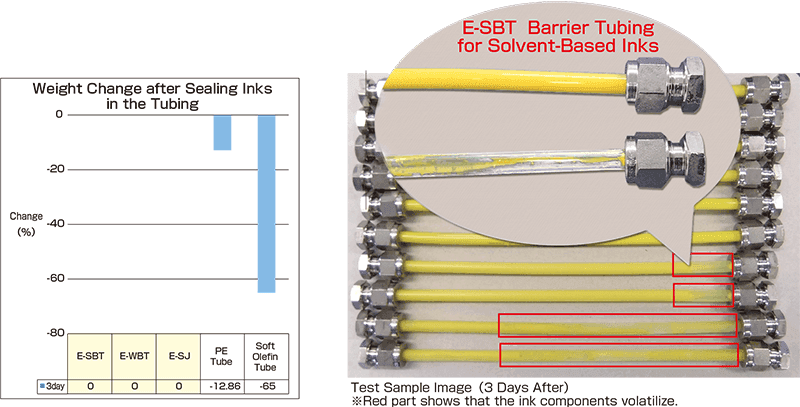## (C) Solvent Barrier Test Data

### 1. Solvent Barrier Test

Seal the solvent (Diethylene Glycol Diethyl Ether) in the tubing.
Then, leave the tubing under the condition of temperature (60 $^\circ$C) with sealing both ends.
Measure the weight (weight changes) of the ink tubing in 7 and 14 days.
*Except the weights of tubing and sealing items, we only measured the weight changes of the solvents.

### 2. Sample

• E-SBT-4 $\times$ 6
• E-SJ-4 $\times$ 6
• Fluorine Rubber Tubing

### 3. Results## (D-1) Slide Curvature Test Data Part I

### 1. Test Methods

Test Device：Slide Curvature Test Machine (Pictures Shown Below)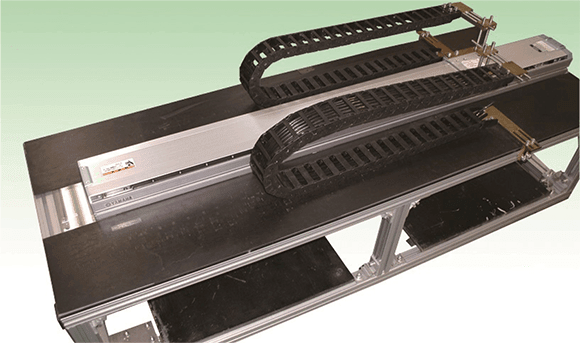• Bending Radius: R = 100
(Inside Energy Chain)
• Test Machine Velocity：810mm/sec
• Number of Attempts：5 Million Times
• Test Temperature：Room Temperature (20 – 30 $^\circ$C)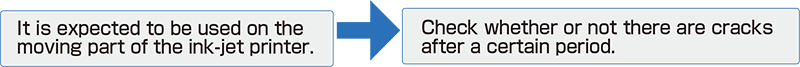### 2. Sample

Olefin-Based (for Water-Based Inks) Tubing

• E-WBT-4 $\times$ 6

Fluorine-Based (for Solvent-Based Inks) Tubing

• E-SBT-4 $\times$ 6
• E-SJ-4 $\times$ 6
• E-SJUS-4 $\times$ 6

### 3. Results

No Cracks or Tears Found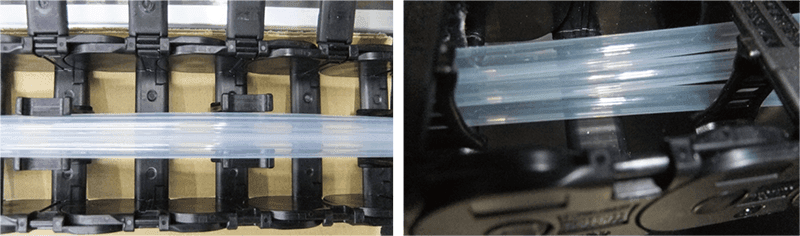## (D-2) Slide Curvature Test Data Part II

Sample A (Flexible Fluorine (ETFE) Resin Tubing; I.D. 4 mm $\times$ O.D. 6 mm) and Sample B (Olefin Tube manufactured by other companies; I.D. 4 mm $\times$ O.D. 6 mm) are installed under the slide curvature test machine. In a state where the bending radius is 50 mm, the sample conditions (1 million times after the friction between the tubes and the cable tube when the continuous slide bending is performed) are measured.

Test Device：Slide Curvature Test Machine (Pictures Shown Below)• Bending Radius: R = 100
(Inside Energy Chain)
• Test Machine Velocity：810mm/sec
• Number of Attempts：1 Million Times
• Test Temperature：Room Temperature (20 – 30 $^\circ$C)

### Results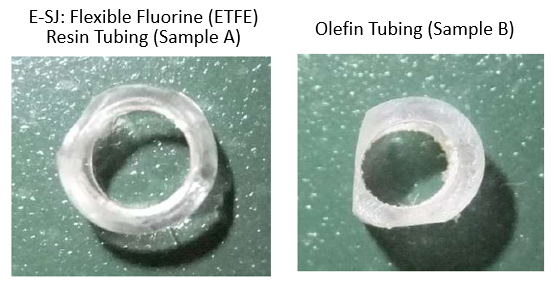The photographs above are the samples after the slide curvature test for 1 million times. As shown in the photographs above, it was confirmed that Sample B was shaved deeper than Sample A. In the most scraped portion (thickness), Sample B was scraped more than 2 times as much as Sample A.

## (D-3) Slide Curvature Test Data Part III

Sample A (PFA Tubing: I.D. 4mm $\times$ O.D. 6mm; manufactured by other companies), Sample B (Flexible Fluorine (ETFE) Resin Tubing Black: E-SJ-4$\times$6-BK) and Sample C (Flexible Fluorine (ETFE) Resin Tubing Black (Ultra Soft): E-SJUS-4$\times$6-BK) are installed under the slide curvature test machine. In a state where the bending radius is 50 mm, the sample conditions (5 million times after the friction between the tubes and the cable tube when the continuous slide bending is performed) are measured.

Test Device：Slide Curvature Test Machine (Pictures Shown Below)• Bending Radius: R = 50
(Inside Energy Chain)
• Test Machine Velocity：810mm/sec
• Number of Attempts：5 Million Times
• Test Temperature：Room Temperature (20 – 30 $^\circ$C)

### Results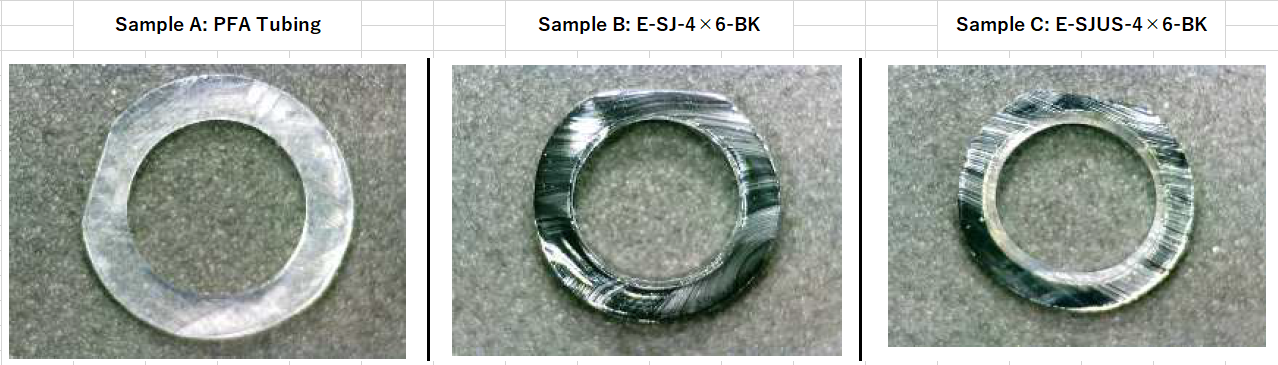The photographs above are the samples after the slide curvature test for 5 million times. As shown in the photographs above, it was confirmed that Sample A was shaved deeper than Sample B and Sample C.

## (E) Flexibility Test Data

### 1. Test Methods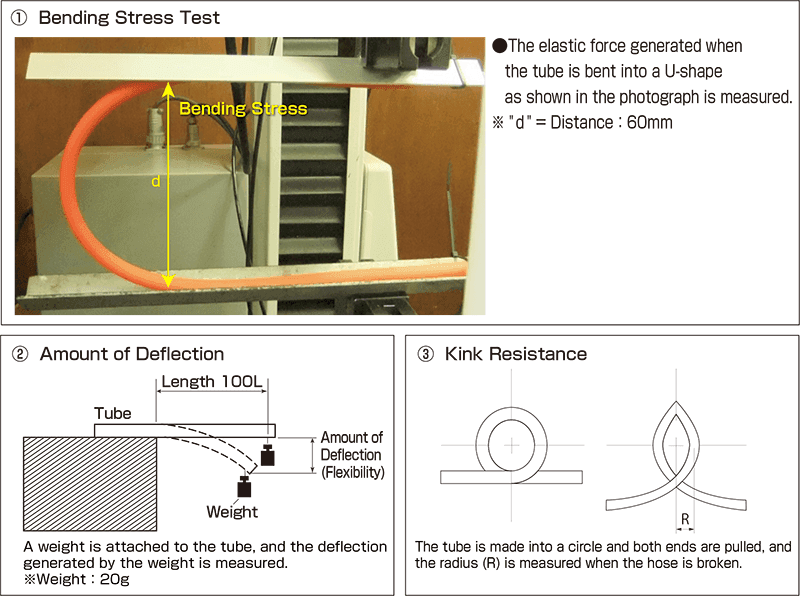### 2. Results

Compared with tubes manufactured by other corporations, HAKKO tubes show higher bending stress and larger amount of deflection, enabling space-saving installation.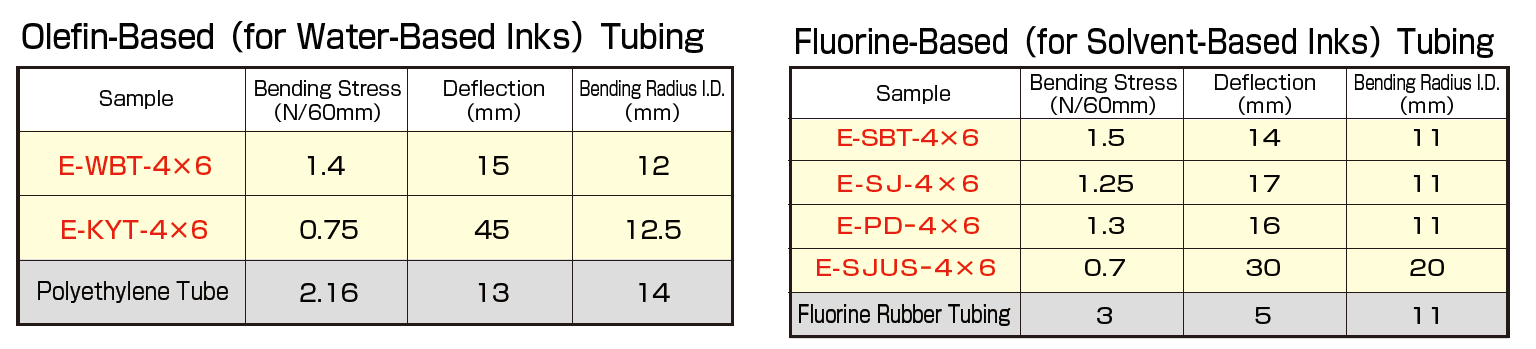*Please note that in terms of bending stress value, “smaller bending stress value” means “more softness.”

## (F) Ultraviolet Penetration Test Data

### 1. Ultraviolet Penetration Data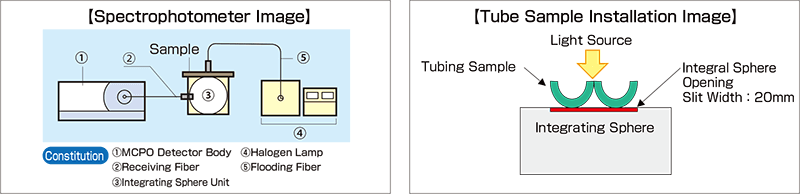### 2. Sample

• Barrier Tubing Black for Solvent-Based Ink (E-SBT-4 $\times$ 6-UV)
• Barrier Tubing Black for Water-Based Ink (E-WBT-4 $\times$ 6-UV)
• Flexible Fluorine (ETFE) Resin Tubing Black (E-SJ-4 $\times$ 6ｰBK)
• Flexible Fluorine (ETFE) Resin Tubing Black (Ultra Soft Type) (E-SJUS-4 $\times$ 6ｰBK)

### 3. Results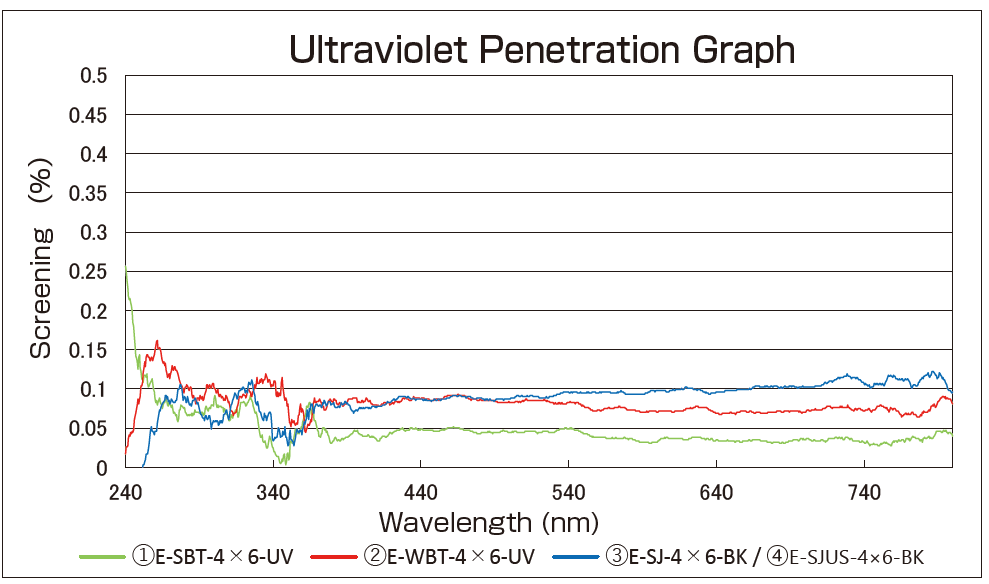Ultraviolet Screening
99% or over

## Other Test Data

Besides the test data above, we can conduct the following tests:

• (a) Burst Pressure Test by Temperature
• (b) Delamination Strength Test (Inner Layer vs. Middle Layer)
• (c) Outer Layer Smoothness Test Data
• (d) Heat Shrink Test
• (e) Pull-Off Strength Test from the Fittings
• (f) Fitting Insertion Test
• (g) Contact Angle Test (Inner Layer Material)

If you are interested in one of these test data, please consult with us from here.